## Introduction

This tutorial was presented at the NGCM Summer Academy 2016 Basics B course, and is created for the purpose of teaching Python programming concepts to extend that of the Software Carpentry style basics course. The material is taught in Python 3, and code outputs displayed are the result from a Python 3.5 Jupyter notebook.

The concepts of OOP are often covered lightly in undergraduate and basics courses. Despite this, Large amounts of scientific Python packages are written in an object oriented manner. The skills learned in this workshop should therefore enable scientists to write (and more importantly, read) the packages they are using, and also serve as a starting platform for those wanting to learn more advanced OOP languages such as C++ or Java.## Software Requirements

Setup instructions for a range of users are available here. Click here for more info on how to start a live Jupyter notebook.

# Getting Started

Teaching material is largely in Jupyter Notebook format, and should be downloaded or cloned from GitHub - data included in the links will be necessary for completing the exercises.

## Intermediate Python¶

Paul Chambers, Ioannis Begleris

P.R.Chambers@soton.ac.uk## Material Outline¶

• Basics Revision

• Lists, loops, functions, Numpy, Matplotlib
• Tuples, mutability

• Dictionaries

• Associative data, function argument unpacking

## Material Outline (ctd)¶

• Object Oriented Programming
• Classes
• Initialization and __init__, self
• Encapsulation
• Inheritance
• Magic operators

## Prerequisites¶

If you are following this course and do not know how to obtain the above requirements, see Setup Instructions.

In :
# Run this cell before trying examples
import numpy as np
import matplotlib.pyplot as plt
%matplotlib inline


## Basics Refresher¶

• Lists
• For loops
• Functions
• Numpy
• Matplotlib

### External Material¶

• If you missed the basics, try these:

### Lists¶

• Mutable Container
• Often appended to in a loop with 'append'
• Handles mixed data types
• Allows indexing and slicing

#### Example: List indexing and slicing¶

In :
lists = [10, 12, 14, 16, 18]
print(lists)      # Index starts at 0

print(lists[-1])     # Last index at -1

print(lists[0:3])    # Slicing: exclusive of end value
#  i.e. get i=(0, 1, .. n-1)

print(lists[3:])     # "slice from i=3 to end"

10
18
[10, 12, 14]
[16, 18]


#### Example: Methods of lists¶

In :
# List construction example
a = []
print(a)

a.append('Hello world')
print(a)

[]
['Hello world']

In :
a.extend([1, 2, 3, 4])
print(a)

a.remove(1)            # Remove value 1 from a
print(a)

a.pop(0)
print(a)

['Hello world', 1, 2, 3, 4]
['Hello world', 2, 3, 4]
[2, 3, 4]

In :
# All methods
# a.            # Tab complete behaviour?


### For Loops¶

• Need to iterate through something in Python
• Not an explicit counting loop as used in lower level languages
• Syntax (var is user defined) -
  For var in iterable:

#### Basic Example:¶

In :
powers = [0, 1, 2, 3]
for power in powers:
value = 10 ** (power)
print("10 to the power of {} is {}".format(power, value))

10 to the power of 0 is 1
10 to the power of 1 is 10
10 to the power of 2 is 100
10 to the power of 3 is 1000

In :
# Better to use Pythons built in 'range' here:
for i in range(4):
print("10 to the power of {} is {}".format(i, 10**i))

10 to the power of 0 is 1
10 to the power of 1 is 10
10 to the power of 2 is 100
10 to the power of 3 is 1000


#### List compehension¶

In :
x = [i**2 for i in range(10)]
y = [i*10 for i in range(10)]
print(x)
print(y)

[0, 1, 4, 9, 16, 25, 36, 49, 64, 81]
[0, 10, 20, 30, 40, 50, 60, 70, 80, 90]

In :
#### How would you create a list from zero to 100 in increments of 5 in one line


### Functions¶

• def followed by function name and parameters in a parenthesis
• return output_var(s) ends the function
• Args parsed by assignment: Mutability dictates whether the function can update args directly

#### Basic function¶

In :
def square_root(x):
"""Useful docstring: Calculates and returns square root of x"""
i = x ** 0.5
return i

x = 10
y = square_root(x)
print('The square root of {} is {}'.format(x, y))

The square root of 10 is 3.1622776601683795

In :
# We can set a default value to the function
def square_root(x=20):
i = x ** 0.5
return i

print(square_root())

4.47213595499958

In :
# Loops, functions and appending
mylist = []
for i in range(1,5):
mylist.append(square_root(i))
print(mylist)

[1.0, 1.4142135623730951, 1.7320508075688772, 2.0]


#### Example: Arguments and mutability¶

In :
def update_integer(i):
# attempt to update i (integers) are immutable
i += 1

def update_list_end(arglist):
arglist[-1] = 50   # Lists are mutable: updates args directly!

a = 1
update_integer(a)
print(a)

mylist = [0, 1, 2, 3, 4]
update_list_end(mylist)
print(mylist)

1
[0, 1, 2, 3, 50]


Note above that there is no return statement required: implicitly this function will return the Python builtin-in value None.

### Numpy¶

• Arrays and array operations
• Mathematical evaluations - fast on np.array
• Linear algebra
• Useful functions
• Allows integration between C/C++ and Fortran

#### Examples: Basic functions¶

In :
import numpy as np

# basic usage: arange, linspace, array ops
x = np.linspace(0, 10, 11)    # use 11 points
print(x)

y = np.arange(0, 10, 1)       # use step size of 1
print(y)

print('The average of x is', np.average(x))

[  0.   1.   2.   3.   4.   5.   6.   7.   8.   9.  10.]
[0 1 2 3 4 5 6 7 8 9]
The average of x is 5.0


#### Example: 2D arrays¶

In :
M1 = np.array([[2,3],[6,3]])
M2 = np.array([[5,6],[2,9]])

print('M1:')
print(M1)

print('M2:')
print(M2)

M1:
[[2 3]
[6 3]]
M2:
[[5 6]
[2 9]]

$$M_1 = \begin{bmatrix} 2 & 3 \\ 6 & 3\end{bmatrix}, \quad M_2 = \begin{bmatrix} 5 & 6 \\ 2 & 9\end{bmatrix}$$
In :
M3 = M1 * M2            # Element-wise multiplication
print(M3, '\n')

M4 = np.dot(M1, M2)     # Matrix multiplication
print(M4)

[[10 18]
[12 27]]

[[16 39]
[36 63]]

In :
# Given array [0, np.pi/2., np.pi, 3*np.pi/4.] what would you
# expect passing it to np.sin ????

In :
# live coding show some numpy functions.


### Matplotlib¶

• Popular plotting library
• Can produce publication quality plots
• Allows embedded LaTeX formatting

#### Example: 2D plotting¶

In :
x = np.linspace(0, 2*np.pi)
y = np.sin(x)

fig = plt.figure(figsize=(12, 5))
ax.plot(x, y,'o-')
ax.margins(0.1)
ax.set_title('2D plot')
ax.set_xlabel('$x$')
ax.set_ylabel(r'$sin(x)$')

Out:
<matplotlib.text.Text at 0x7f595ff23a58>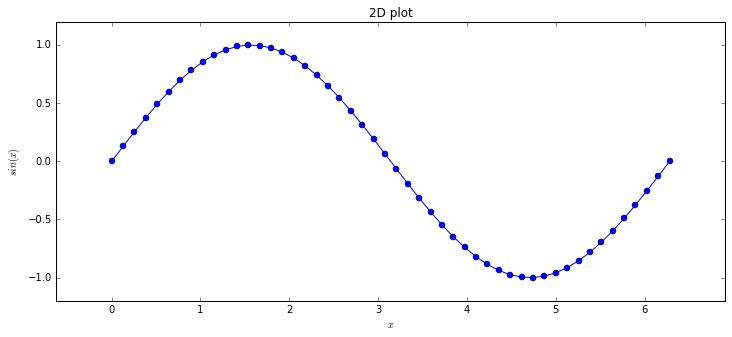#### What if we want increments of $\pi$ on our x axis¶

In :
xtick_values = np.linspace(0, 2*np.pi, 5)
xtick_labels = ['$0$', r'$\frac{\pi}{2}$', r'$\pi$', r'$\frac{3\pi}{2}$',
r'$2\pi$']
fig = plt.figure(figsize=(12, 5))
ax.set_title('2D plot')
ax.margins(0.1)
ax.set_xlabel('$x$');  ax.set_ylabel(r'$sin(x)$')
ax.set_xticks(xtick_values)
ax.set_xticklabels(xtick_labels, fontsize=25);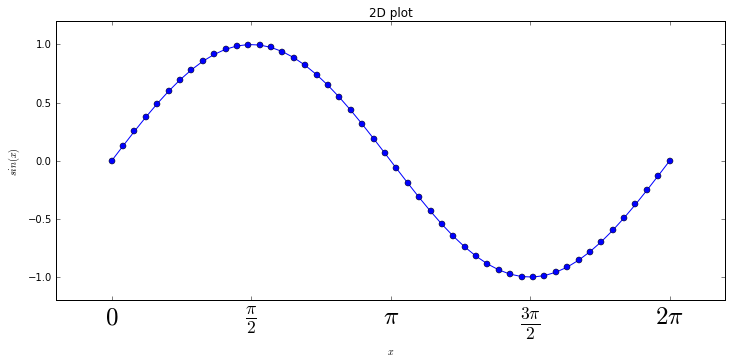#### Example: 3D plot¶

In :
x = np.linspace(-1, 1, 101)
y = np.linspace(-1, 1, 101)
X, Y = np.meshgrid(x, y)
Z = np.sin(X + Y)**2

In :
from mpl_toolkits.mplot3d import Axes3D
fig = plt.figure(figsize=(12, 5))
surf = ax.plot_surface(X, Y, Z)
ax.set_xlabel(r'$X$')
ax.set_ylabel(r'$Y$')
ax.set_zlabel(r'$Z$')

plt.show()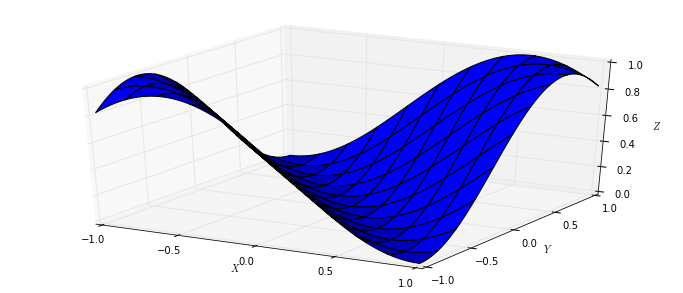In :
# Live coding Bay....


## Exercise: Basics Refresher¶

Python Basics Refresher

To the top

## Tuples¶

• An Immutable List
• Faster than a List (fixed memory)
• Useful for structured data
• No append method - bad for sequential data

#### Example: Tuple Syntax¶

In :
# Create a 'Name, Age' Tuple  using bracket notation
my_tuple = ('Dave', 42)

print(type(my_tuple))
print(my_tuple)

<class 'tuple'>
('Dave', 42)

In :
# Create Tuple using bracket-less notation
my_tuple2 = 'Bob', 24

print(type(my_tuple2))
print(my_tuple2)

<class 'tuple'>
('Bob', 24)


#### Example: Usage¶

In :
# Tuple indexing
my_tuple = ('Dave', 42)
print(my_tuple)
print(my_tuple)

Dave
42

In :
# Could make a list of tuples:
tups = [('Dave', 42), ('Bob', '24')]
# ... and then iterate over it
for tup in tups:
print("{} is {} years old".format(tup, tup))

Dave is 42 years old
Bob is 24 years old


#### Example: Tuple Unpacking¶

In :
# Store multiple variables using tuples:
my_tuple = 'Dave', 42
a, b = my_tuple

print('a = {}'.format(a))
print('b = {}'.format(b))

a = Dave
b = 42

In :
# Swap Variables using tuples:
b, a = a, b

print('a = {}'.format(a))
print('b = {}'.format(b))

a = 42
b = Dave


#### Example: When NOT to use a Tuple (1)¶

In :
# extending or overwriting contents
my_tuple = 'Dave', 42

# my_tuple = 'Steve'     # Will give an error


#### Example: When NOT to use a Tuple (2)¶

In :
# Sequences: Stick with a list
seq = []   # tuples have no append method, so need a list []
for i in range(10):
seq.append(i**2)

print(seq)

[0, 1, 4, 9, 16, 25, 36, 49, 64, 81]

In :
# Or a numpy array:
print(np.arange(10)**2)

[ 0  1  4  9 16 25 36 49 64 81]

In :
# Create a tuple of lists 'a' - can you change the values in
# the lists?

In :
# Live coding Bay....


## Dictionaries¶

• Set of key : value pairs
• Ordering follows hash table rules, not so intuitive to humans
• Use curly braces - {} or dict keyword

#### Example: Fruit Prices Lookup Table - Construction¶

In :
# Using the dict function:
fruit = [('apples', 2), ('bananas', 5), ('pears', 10)]
price_table = dict(fruit)
print(price_table)

{'pears': 10, 'apples': 2, 'bananas': 5}

In :
# Short hand (Arguably neater)
price_table = {'apples': 2, 'pears': 10, 'bananas': 5}
print(price_table)

{'pears': 10, 'apples': 2, 'bananas': 5}


Note: notice that the consistent order on printing of the dictionaries, even though the inputs are reordered. The ordering of hash tables is well defined, but not in a human-intuitive sense. We should therefore treat the data as if it was unordered.

#### Example: Accessing values from keys¶

In :
price_table = {'apples': 2, 'bananas': 5, 'pears': 10}

akey = 'apples'
print("The price of {} is {}p".format(akey, price_table[akey]))

The price of apples is 2p


#### Example: Iterating over a dictionary¶

In :
# Iterating over the dictionary will iterate over its keys
price_table = {'apples': 2, 'bananas': 5, 'pears': 10}

for key in price_table:
print("{} cost {}p".format(key, price_table[key]))

pears cost 10p
apples cost 2p
bananas cost 5p

In :
# Or use the items method:
for key, val in price_table.items():
print("{} cost {}p".format(key, val))

pears cost 10p
apples cost 2p
bananas cost 5p


#### Example: Shopping list using dictionary price lookup¶

In :
# I don't like pears, so let's buy apples and bananas
shopping_list = [('apples', 50), ('bananas', 20)]
total = 0
for item, quantity in shopping_list:
price = price_table[item]
print('Adding {} {} at {}p each'.format(quantity, item, price))
total += price * quantity

print(total)

Adding 50 apples at 2p each
Adding 20 bananas at 5p each
200


#### Example: When NOT to use a Dictionary¶

In :
# Hoping for ordered data:
alpha_num = {'a': 0, 'b': 1, 'c': 2}

for i, key in enumerate(alpha_num.keys()):
print("{} has a value of {}".format(key, i))   # This is wrong

b has a value of 0
c has a value of 1
a has a value of 2


#### Example: Dictionary unpacking using '**'¶

In :
mydict = {'a':1, 'b':2, 'c':3}

def myFunc(a,b,c):
return a*2, b*2, c*2

myFunc(**mydict)

Out:
(2, 4, 6)
In :
# Live coding Bay....


## Exercise: Tuples and Dictionaries¶

Tuples and Dictionaries Exercise

To the top

## Structured data: Numpy dtypes¶

• Not a class, but motivational example
• Structured associative data
• Multiple data accessible from a single data type
• Identifiers to indicate data type

In :
with open('data/structured_data.txt', 'w') as f:
f.write('#Name    Height    Weight\n')
f.write('John     180    80.5\n')
f.write('Paul     172    75.1\n')
f.write('George   185    78.6\n')
f.write('Ringo    170    76.5\n')

In :
# Notice that the argument is a list of tuples
dt = np.dtype([('Name', np.str_, 16), ('Height', np.int32),
('Weight', np.float64)])

print(data)

[("b'John'", 180, 80.5) ("b'Paul'", 172, 75.1) ("b'George'", 185, 78.6)
("b'Ringo'", 170, 76.5)]

In :
print(data['Name'])
print("{} has weight {}".format(data['Name'], data['Weight']))

["b'John'" "b'Paul'" "b'George'" "b'Ringo'"]
b'John' has weight 80.5

In :
# Live coding Bay....


## Exercise: Numpy dtypes¶

Exercise: Load image data with dtype structured array

• Data is structured, but not elegant
• no methods etc

To the top

# Intro to Python OOP¶

#### (For The Classy Programmer)¶

"Object-oriented programming (OOP) refers to a type of computer programming in which programmers define not only the data type of a data structure, but also the types of operations (functions) that can be applied to the data structure."

Source: Webopedia

## Why OOP?¶

• Naturally structured data
• Functions used in context
• Reduce duplicate code
• Maintainability in large codes/software
• Many other reasons

### OOP in Scientific Computing¶

• Java, C++ and Python designed for OOP
• Everything in Python is an object
• Scientific libraries, visualisation tools etc.
• Pseudo Object Orientation in C and Fortran

### What will I learn about OOP here?¶

• Language in OOP is very different
• Learn language used in eg. C++, Java
• Ability to read code is essential
• Write/migrate code for community library
• Better world! Work recognition etc...

### OOP: Four Fundamental Concepts¶

• Inheritance

• Reuse code by deriving from existing classes
• Encapsulation

• Data hiding

### OOP: Four Fundamental Concepts (2)¶

• Abstraction

• Simplified representation of complexity
• Polymorphism

• API performs differently based on data type

Note: Encapsulation is sometimes also used in OOP to describe the grouping of data with methods. It is however more common for texts to use it to describe the hiding of data as will be done here.

Useful explanations of these concepts for Python can also be found here

## Classes: Basics¶

• Attributes (data)
• Methods (Functions operating on the attributes)
• 'First class citizens': Same rights as core types
• pass to functions, store as variables etc.

#### Example: Numpy arrays showing how it's done¶

In :
# Numpy arrays are classes
import numpy as np
a = np.array([0, 1, 6, 8, 12])
print(a.__class__)
print(type(a))

<class 'numpy.ndarray'>
<class 'numpy.ndarray'>

In :
# We want to operate on the array: try numpy cumulative sum function
print(np.cumsum(a))

[ 0  1  7 15 27]

In :
# np.cumsum('helloworld')  # Should we expect this to work?


#### Example: Numpy arrays showing how it's done (ctd.)¶

• We only know what a cumulative sum means for a narrow scope of data types

• Group them together with an object!

In :
# cumsum is a method belonging to a
a.cumsum()

Out:
array([ 0,  1,  7, 15, 27])

#### Example: Simple class¶

• For now, assume all classes defined by: class ClassName(object)
In :
class Greeter(object):
def hello(self):         # Method (more on 'self' later)
print("Hello World")

agreeter = Greeter()         # 'Instantiate' the class
print(agreeter)
# agreeter.                  # Tab complete?

<__main__.Greeter object at 0x7f595ddcef60>


There's a few things here which I haven't introduced, but all will become clear in the remainder of this workshop.

In :
# Note that we don't pass an argument to hello!
agreeter.hello()

Hello World


## Classes: Initialisation and self¶

• __init__ class method
• Called on creation of an instance
• Convention: self = instance
• Implicit passing of self, explicit receive

Note: Passing of self is done implicitly in other languages e.g. C++ and Java, and proponents of those languages may argue that this is better. "Explicit is better than implicit" is simply the python way.

### What is an "instance"?¶

• Class is like a type
• Instance is a specific realisation of that type
• eg. "Hello World" is an instance of string
• Instances attributes are not shared

### Classes: Initialisation vs Construction¶

• Initialisation changes the instance when it is made
• ... __init__ is not technically Construction (see: C++)
• __new__ 'constructs' the instance before __init__
• __init__ then initialises the content

More info: The Constructor creates the instance, and the Initialiser Initialises its contents. Most languages e.g. C++ refer to these interchangably and perform these steps together, however the new style classes in Python splits the process.

The difference is quite fine, and for most purposes we do not need to redefine the behaviour of __new__. This is discussed in several Stack Overflow threads, e.g.

#### Example: Class Initialisation¶

In :
class A(object):
def __init__(self):
print("Hello")

a_instance = A()
print(type(a_instance))

Hello
<class '__main__.A'>


### Instance attributes and Class attributes¶

• Instance attributes definition: self.attribute = value
• Class attributes defined outside functions (class scope)
• Class attributes are shared by all instances
• Be careful with class attributes

#### Example: Defining Instance attributes/methods¶

In :
class Container(object):
"""Simple container which stores an array as an instance attribute
and an instance method"""
def __init__(self, N):
self.data = np.linspace(0, 1, N)

def plot(self):
fig = plt.figure()
ax.plot(self.data, 'bx')

mydata = Container(11)      # 11 is passed as 'N' to __init__
print(mydata.__dict__)      # __dict__ is where the attr: value
#  pairs are stored!
mydata.plot()

{'data': array([ 0. ,  0.1,  0.2,  0.3,  0.4,  0.5,  0.6,  0.7,  0.8,  0.9,  1. ])}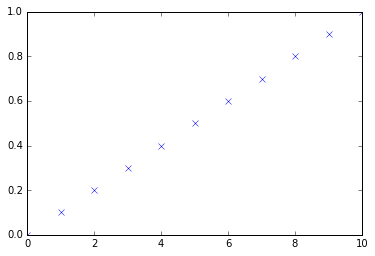• Implement a class which takes an input number 'N', and doubles and stores it as an instance attribute
• Test it!
In :
# Code solution here:


#### Example: Class attributes vs instance attributes¶

In :
class Container(object):
data = np.linspace(0, 1, 5)    # class attribute
def __init__(self):
pass

a, b = Container(), Container()
print(a.data)
print(b.data)

[ 0.    0.25  0.5   0.75  1.  ]
[ 0.    0.25  0.5   0.75  1.  ]

In :
a.data = 0                     # Creates INSTANCE attribute
Container.data = 100           # Overwrites CLASS attribute
print(a.data)
print(b.data)

0
100


### Class vs Instance attributes: priority¶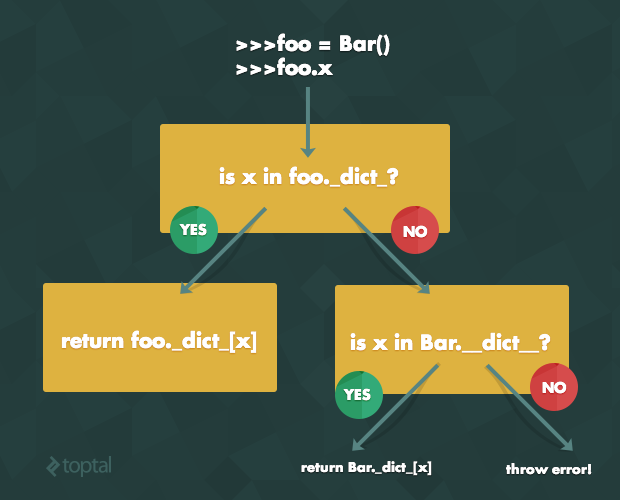Note: There's a couple of things going on in this example which are worth elaborating on. By specifying ClassName.attribute, in this case Container.data = 100 we've overwritten the value of data that EVERY instance of the Container class will access. Hence printing b.data gives the expected result.

By setting a.data at the same time, we have set an instance attribute, which is given priority and called first even though we overwrote the class attribute after assigning this.

This could create a hard to track bug. To avoid it:

• Stick to instance variables unless you specifically need to share data e.g. constants, total number or list of things that are shared
• Don't overwrite things you know are class attributes with instance.attr unless you really know what you're doing (even then, it's probably better and more readable to make it an instance attribute)

For a really in depth explanation of class vs instance attributes, see either of the following links:

#### Example: Implicit vs Explicit passing Instance¶

In :
class Container(object):
def __init__(self, N):
self.data = np.linspace(0, 1, N)

def print_data(self):
print(self.data)

a = Container(11)

a.print_data()          # <<< This is better
Container.print_data(a)

[ 0.   0.1  0.2  0.3  0.4  0.5  0.6  0.7  0.8  0.9  1. ]
[ 0.   0.1  0.2  0.3  0.4  0.5  0.6  0.7  0.8  0.9  1. ]


## Exercise: Basics and Initialisation¶

Python OOP 1 Basics and Initialisation with images

To the top

## Classes: Encapsulation¶

• Hiding data from users (and developers)
• Use underscores '_' or '__'
• Useful if data changing should be controlled
• Convention only in Python - not enforced!

### Single vs Double Underscore¶

• Single underscore

• Nobody outside this class or derived classes should access or change
• Double underscore

• Stronger attempt to enforce the above
• Also 'mangles' the attribute name with

instance._ClassName__Attribute

#### Example: Data hiding "protected", single underscore¶

In :
class Fruit(object):
def __init__(self):
self._hasjuice = True

def juice(self):
if not self.isfull(): raise ValueError('No juice!')
self._hasjuice = False

def isfull(self):
return self._hasjuice

orange = Fruit()
print(orange.isfull())
orange.juice()
print(orange.isfull())

True
False

In :
# orange.                  # tab completion behaviour?
# orange._                 # tab completion behaviour now?
orange.isfull()

Out:
True

#### Example: Data hiding "private", double underscore¶

In :
class Fruit(object):
def __init__(self):
self.__hasjuice = True

def juice(self):
if not self.isfull(): raise ValueError('No juice!')
self.__hasjuice = False

def isfull(self):
return self.__hasjuice

apple = Fruit()

In :
# apple._                       # tab completion behaviour?
apple.juice()
apple._Fruit__hasjuice = False   # Definitely bad!
apple.isfull()

Out:
False

Note: This behaviour can be over used in Python. Programmers from C++ or Java backgrounds may want to make all data hidden or private and access the data with 'getter' or 'setter' functions, however it's generally accepted by Python programmers that getters and setters are unnecessary. The Pythonista phrase is "we are all consenting adults here", meaning you should trust the programmer to interact with your classes and they should trust you to document/indicate which parts of the data not to touch unless they know what they're doing (hence the underscore convention). See the top answer on this Stack Overflow thread.

For an entertaining view of encapsulation, see this blog

In :
# Live coding Bay....


## Classes: Inheritance¶

• Group multiple objects and methods
• Child/Derived class inherits from Parent/Base
• Reduce duplicate code
• Maintanable: changes to base falls through to all
• Beware multiple inheritance rules - we won't cover this

#### Example: Simple inheritance¶

In :
class Parent(object):
# Note the base __init__ is overridden in
# Child class
def __init__(self):
pass
def double(self):
return self.data*2

class Child(Parent):
def __init__(self, data):
self.data = data

achild = Child(np.array([0, 1, 5, 10]))
achild.double()

Out:
array([ 0,  2, 10, 20])

#### Example: Calling parent methods with super¶

In :
class Plottable(object):
def __init__(self, data):
self.data = data
def plot(self, ax):
ax.plot(self.data)

class SinWave(Plottable):
def __init__(self):
super().__init__(
np.sin(np.linspace(0, np.pi*2, 101)))

class CosWave(Plottable):
def __init__(self):
super().__init__(
np.cos(np.linspace(0, np.pi*2, 101)))

fig = plt.figure()
mysin = SinWave();   mycos = CosWave()
mysin.plot(ax);      mycos.plot(ax)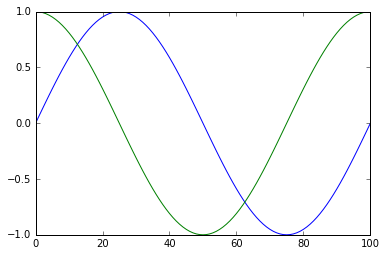Notes:

• We didn't need any arguments to super here as Python 3 allows this
• super requires additional arguments in Python 2, e.g.

super(Class, self).method(args...)

If you were wondering why we should use super().method instead of BaseClass.method, other than the convenience of renaming classes, it relates to multiple inheritance which is beyond the scope of this course. If you need to write programs with multiple inheritance (and there are strong arguments against this), you may want to look at this blog for advanced use of super.

## Classes: Magic Methods¶

• The workhorse of how things 'just work' in Python
• object & builtin types come with many of these
• Surrounded by double underscores
• We've seen one already: __init__!

#### Example: The magic methods of object¶

In :
dir(object)

Out:
['__class__',
'__delattr__',
'__dir__',
'__doc__',
'__eq__',
'__format__',
'__ge__',
'__getattribute__',
'__gt__',
'__hash__',
'__init__',
'__le__',
'__lt__',
'__ne__',
'__new__',
'__reduce__',
'__reduce_ex__',
'__repr__',
'__setattr__',
'__sizeof__',
'__str__',
'__subclasshook__']

### Magic Methods: Closer look¶

• __lt__:

• Called when evaluating
  a < b
• __str__

• Called by the print function
• All magics can be overridden

#### Example: overriding __str__ and __lt__ magic methods¶

In :
class Wave(object):
def __init__(self, freq):
self.freq = freq
self._data = np.sin(np.linspace(0, np.pi, 101)
* np.pi*2 * freq)

def __str__(self):
"""RETURNS the string for printing"""
return "Wave frequency: {}".format(self.freq)

def __lt__(self, wave2):
return self.freq < wave2.freq

wav_low = Wave(10)
wav_high = Wave(50)  # A high frequency wave

print(wav_high)
wav_low < wav_high

Wave frequency: 50

Out:
True
In :
# Live coding Bay....


Note: Magic methods are very briefly introduced here. For an extensive overview of magic methods for Python classes, view Rafe Kettlers blog

## Exercise: Inheritance and Magic Methods¶

Python OOP2 Inheritance and Magic Methods using shapes

To the top

## Python 2 vs 3¶

• Dont need to inherit object in Python 3
• New classes inherit from object
• Inheritance behaviour is different
• Old classes removed in Python 3

#### Example: New class default Python 3¶

In :
class OldSyntax:
pass

class NewSyntax(object):  # This means 'inherit from object'
pass

print(type(OldSyntax))    # Would give <type 'classobj'>
# in Python 2
print(type(NewSyntax))

<class 'type'>
<class 'type'>

• Backwards compatibility: inherit object in Py3

Notes: There are other differences affecting classes which we have not included, such as metaclasses and iterator behaviour, but here is a link to a more complete comparison:

## Extra Material¶

• Harder exercise (if time)

• Should test your knowledge from this course

Exercise: Predator Prey

## Summary¶

• Refreshed your basic Python skills
• Python builtins not included in standard basics course
• Tuples, Dictionaries, Generators
• Software data structuring

## Summary (ctd)¶

• Covered the language and concepts for Object Orientation (Transferable!)
• OOP Implementation in Python
• Read the packages you use daily
• Create maintainable packages for yourself and scientific community

# Thank You¶

P.R.Chambers@soton.ac.uk